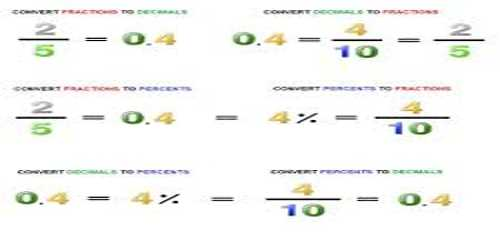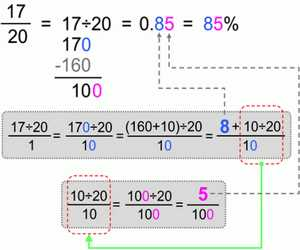Mathematic

# Converting Fraction to Percent and Percent to FractionConverting a Fraction to a Percent

Do the following steps to convert a fraction to a percent:

For example Convert 4/5 to a percent.

• Divide the numerator of the fraction by the denominator (e.g. 4 ÷ 5=0.80)
• Multiply by 100 (Move the decimal point two places to the right) (e.g. 0.80*100 = 80)
• Round the answer to the desired precision.

Remember that a percent is really just a special way of expressing a fraction as a number out of 100. To convert a fraction to a percent, first, divide the numerator by the denominator. Then multiply the decimal by 100.

That is, the fraction 4848 can be converted to decimal by dividing 4 by 8. It can be converted to percent by multiplying the decimal by 100.

4 ÷ 8 = 0.50

0.5 × 100 = 50

So, the fraction 4848 is equivalent to 50%.

On the other hand, when expressed as percentages, these numbers are followed by the % symbol. In this form, the number is compared to a unit, with 100% equivalent to 1. For example, 200% corresponds to 2, and 50% – to 0.5.Converting a Percent to a Fraction

Do the following steps to convert a percent to a fraction:

For example: Convert 83% to a fraction.

• Remove the Percent sign
• Make a fraction with the percent as the numerator and 100 as the denominator (e.g. 83/100)
• Reduce the fraction if needed.
• If you want to express a percentage as a fraction, follow these steps:
• Realize that 100% is equivalent to a unit. That means that every single percent corresponds to 1/100.
• Divide your number by 100%. Let’s assume we want to turn 25% into a fraction. After dividing it by 100%, we will get 25/100.
• Simplify the fraction by finding the greatest common factor. For 25 and 100, the LCD is equal to 25. Hence 25/100 = (25/25) / (100/25) = 1/4.Simple calculations can be done by hand in a few seconds. If you are working with large numbers, we recommend relying on this convert fraction to percent calculator – it automatically simplifies all fractions, making hand calculations unnecessary.

Information Source: